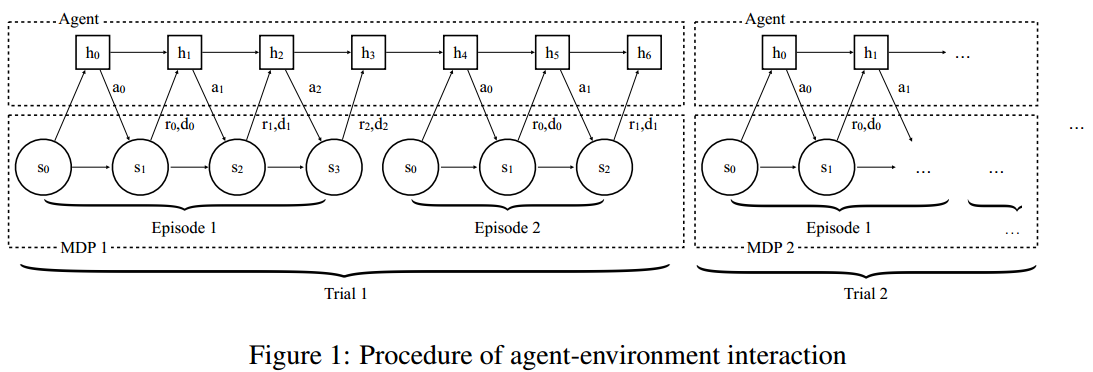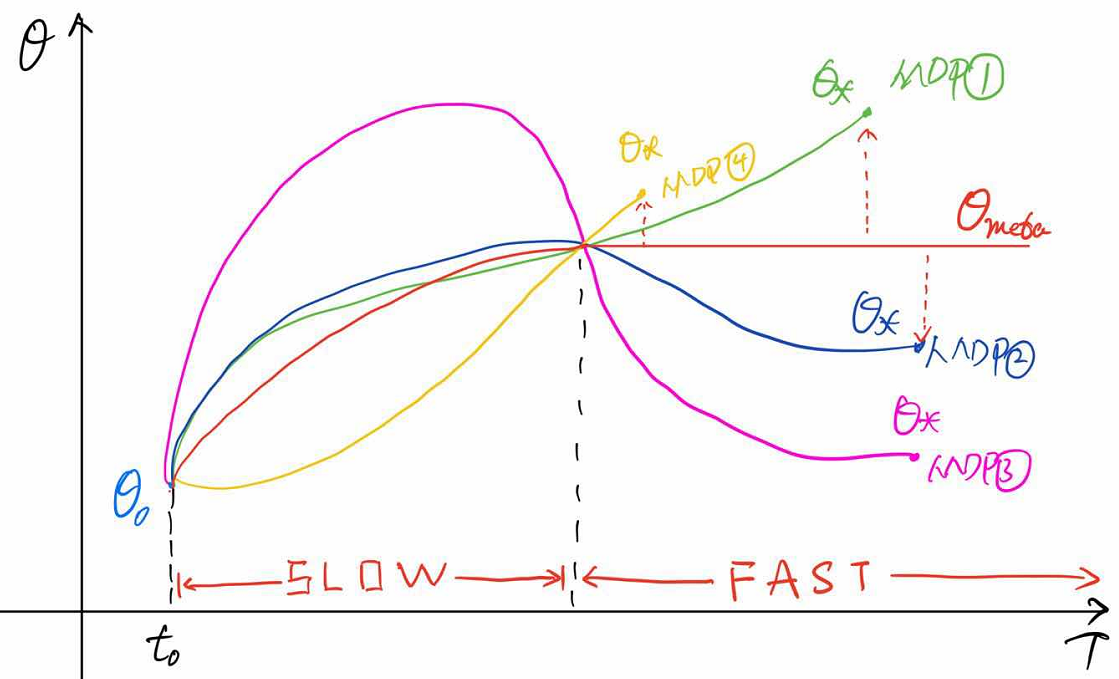• 可以拓宽知识面，了解众家思想
• 我个人认为，这样的元学习并没有达到让机器”学会如何学习的学习方法“的目的，即“learning to learn”

# 简介

• 自动调超参数，学习率等
• 使用分层贝叶斯方法在动力学模型上保持后验，并根据后验应用Thompson采样
• 许多分层强化学习的工作都提出从以前的任务中提取可重用的技能，以加快对新任务的探索

we view the learning process of the agent itself as an objective, which can be optimized using standard reinforcement learning algorithms. The objective is averaged across all possible MDPs according to a specific distribution, which reflects the prior that we would like to distill into the agent.

# 正文• 在这种训练方式下，需要来回切换任务，即切换MDP过程
• 每个trail代表一个MDP过程
• MDP需要从MDPs分布$\rho_{\mathcal{M}} : \mathcal{M} \rightarrow \mathbb{R}_{+}$中采样，至于$\mathbb{R}_{+}$是什么，我不知道
• 在每个trial中训练多个episode，个数用$n$表示，图示表示$n=2$
• $h$代表RNN网络中的知识参数
• 同一个trial中$h$可以传承，但是，当切换MDP问题，即进行下一个trail时，参数$h$需要重新初始化
• 输入不是单纯的状态$s$，而是$\left ( s_{t+1},a_{t},r_{t},d_{t} \right )$，其中，$d_{t}$代表episode结束的标志，输出为动作$a_{t+1}$。（输入往往需要embed为$\phi(s, a, r, d)$）
• 训练过程的目标不是最大化一个episode的累计奖励，而是最大化一个trial的累计奖励

• RNN使用GRUs（Gated Recurrent Units）
• 输入为$\phi(s, a, r, d)$

• TRPO，原因：性能出色，不需要大量调参
• 使用baseline减小方差
• 考虑使用了GAE

• MDP问题：在多臂老虎机和网格MDPs任务中，与理论上合理的算法（没有指出是哪些算法）性能相当
• POMDP问题：图像输入的导航任务中，实验表明，智能体能够有效地利用学习到的视觉信息和以往情景中获得的短期信息。实验结果视频：https://goo.gl/rDDBpb

# 个人见解

（WTF？RNN训练智能体+多任务切换+更改网络输入+任务级目标函数 这就是元强化学习啦？？？）-------------本文结束感谢您的阅读-------------

0%